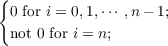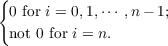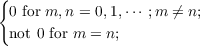#### Vol. 100, No. 2, 1982

 Recent Issues Vol. 311: 1 Vol. 310: 1  2 Vol. 309: 1  2 Vol. 308: 1  2 Vol. 307: 1  2 Vol. 306: 1  2 Vol. 305: 1  2 Vol. 304: 1  2Online Archive Volume: Issue:The Journal Subscriptions Editorial Board Officers Contacts Submission Guidelines Submission Form Policies for Authors ISSN: 1945-5844 (e-only) ISSN: 0030-8730 (print) Special Issues Author Index To Appear Other MSP Journals
Biorthogonal polynomials suggested by the Jacobi polynomials

### H. C. Madhekar and N. K. Thakare

Vol. 100 (1982), No. 2, 417–424
##### Abstract

In this paper we introduce and study a pair of biorthogonal polynomials that are suggested by the classical Jacobi polynomials. Let α > 1, β > 1 and Jn(α,β,k;x) and Kn(α,β,k;x), n = 0,1,2, be respectively the polynomials of degree n in xk and x, where x is real, k is a positive integer such that these two polynomial sets satisfy biorthogonality conditions with respect to the weight function (1 x)α(1 + x)β, namely

 ∫ −11(1 − x)α(1 + x)βJ n(α,β,k;x)xi dx is(1)
and
 ∫ −11(1 − x)α(1 + x)βK n(α,β,k;x)(1 − x)ki dx is(2)
It follows from (1) and (2) that
 ∫ −11(1 − x)α(1 + x)βJ n(α,β,k;x)Km(α,β,k;x)dx is(3)
and conversely.

For k = 1 both these sets are reduced to the Jacobi polynomial sets. We obtain generating functions, recurrence relations for both these sets and explicitly show that they satisfy biorthogonality conditions.

##### Mathematical Subject Classification 2000
Primary: 33A65, 33A65
Secondary: 42C05
##### Milestones